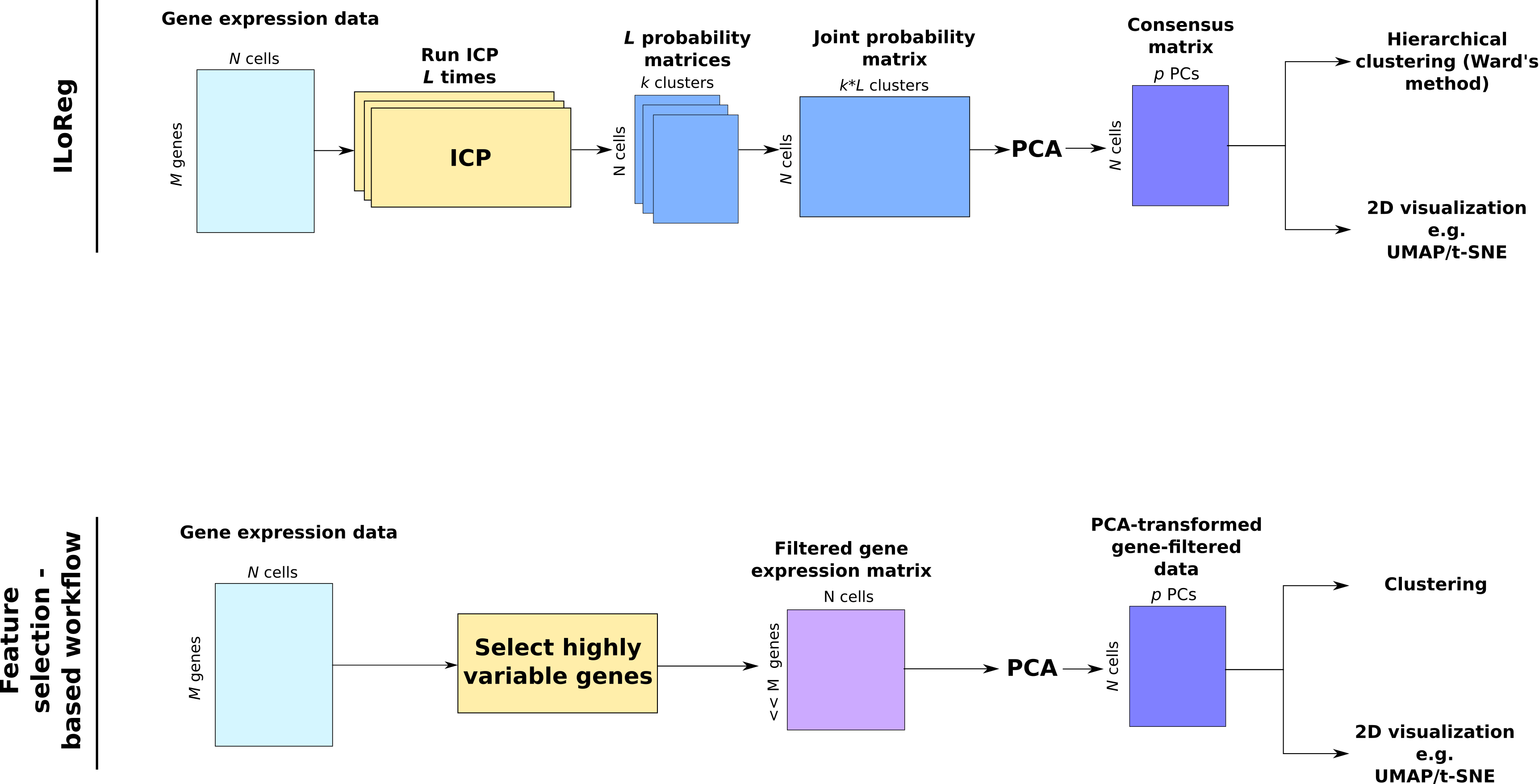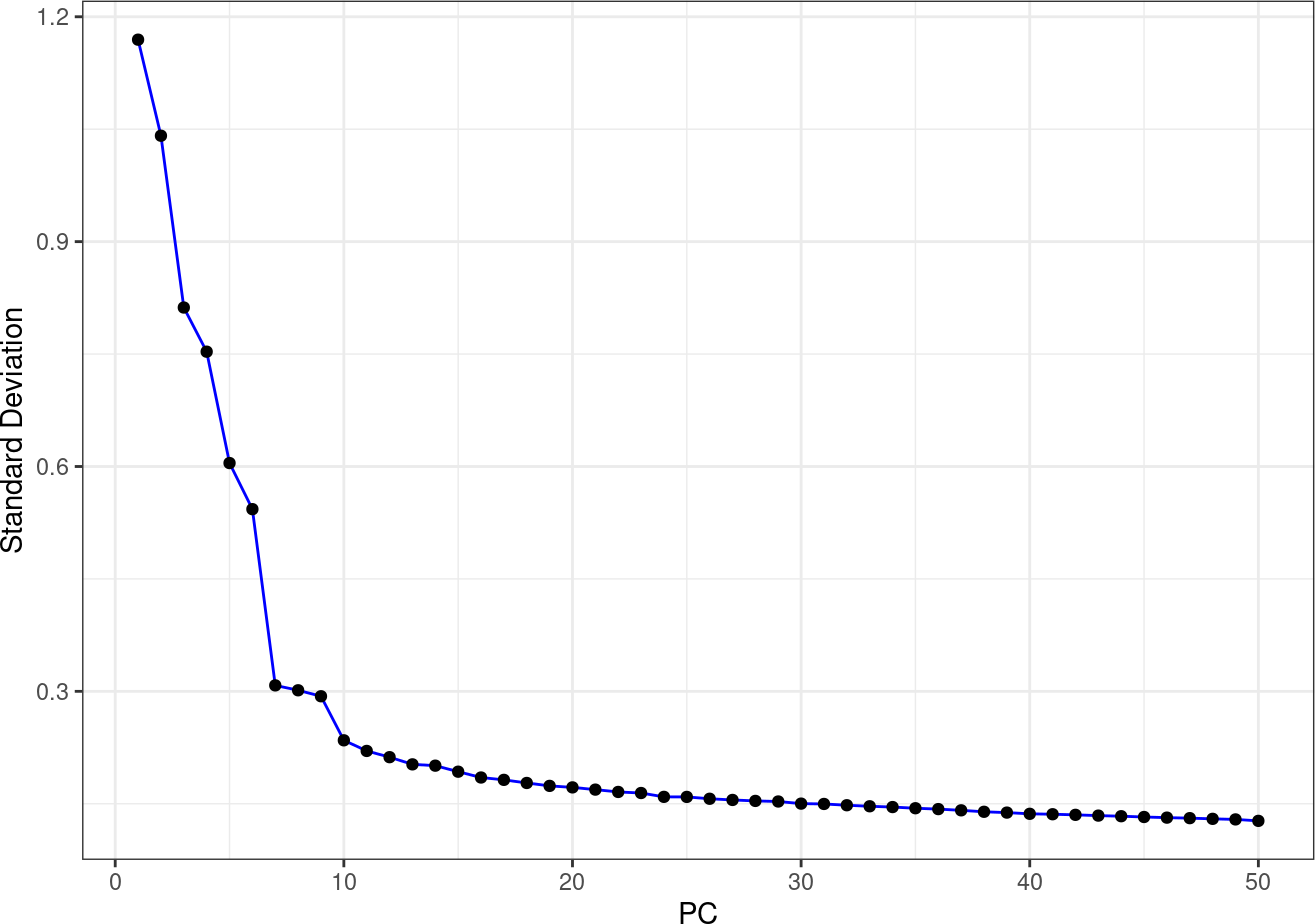# 1 Introduction

ILoReg is a novel tool for cell population identification from single-cell RNA-seq (scRNA-seq) data. In our study , we showed that ILoReg was able to identify, by both unsupervised clustering and visually, rare cell populations that other scRNA-seq data analysis pipelines were unable to identify.

The figure below illustrates the workflows of ILoReg and a typical pipeline that applies feature selection prior to dimensionality reduction by principal component analysis (PCA).Figure: Workflows of ILoReg and a feature-selection based approach.

In contrast to most scRNA-seq data analysis pipelines, ILoReg does not reduce the dimensionality of the gene expression matrix by feature selection. Instead, it performs probabilistic feature extraction using iterative clustering projection (ICP), yielding an $$N \times k$$ -dimensional probability matrix, which contains probabilities of each of the $$N$$ cells belonging to the $$k$$ clusters. ICP is a novel self-supervised learning algorithm that iteratively seeks a clustering with $$k$$ clusters that maximizes the adjusted Rand index (ARI) between the clustering $$C$$ and its projection $$C'$$ by L1-regularized logistic regression. In the ILoReg consensus approach, ICP is run $$L$$ times and the $$L$$ probability matrices are merged into a joint probability matrix and subsequently transformed by principal component analysis (PCA) into a lower dimensional ($$N \times p$$) matrix (consensus matrix). The final clustering step is performed using hierarhical clustering by the Ward’s method, after which the user can extract a clustering with $$K$$ consensus clusters. Two-dimensional visualization is supported using two popular nonlinear dimensionality reduction methods: t-distributed stochastic neighbor embedding (t-SNE) and uniform manifold approximation and projection (UMAP). Additionally, ILoReg provides user-friendly functions that enable identification of differentially expressed (DE) genes and visualization of gene expression.

# 2 Installation

ILoReg can be downloaded from Bioconductor and installed by executing the following command in the R console.

if(!requireNamespace("BiocManager", quietly = TRUE))
install.packages("BiocManager")
BiocManager::install("ILoReg")

# 3 Example: Peripheral Blood Mononuclear Cells

In the following, we go through the different steps of ILoReg’s workflow and demonstrate it using a peripheral blood mononuclear cell (PBMC) dataset. The toy dataset included in the ILoReg R package (pbmc3k_500) contains 500 cells that have been downsampled from the pbmc3k dataset . The preprocessing was rerun with a newer reference genome (GRCh38.p12) and Cell Ranger v2.2.0 .

## 3.1 Setup a SingleCellExperiment object and prepare it for ILoReg analysis

The only required input for ILoReg is a gene expression matrix that has been normalized for the library size, with genes/features in rows and cells/samples in columns. The input can be of matrix, data.frame or dgCMatrix class, which is then transformed into a sparse object of dgCMatrix class. Please note that the method has been designed to work with sparse data, i.e. with a high proportion of zero values. If, for example, the features of your dataset have been standardized, the run time and the memory usage of ILoReg will likely be much higher.

suppressMessages(library(ILoReg))
suppressMessages(library(SingleCellExperiment))
suppressMessages(library(cowplot))
# The dataset was normalized using the LogNormalize method from the Seurat R package.
sce <- SingleCellExperiment(assays = list(logcounts = pbmc3k_500))
sce <- PrepareILoReg(sce)
## Data in logcounts slot already of dgCMatrix class...
## 13865/13865 genes remain after filtering genes with only zero values.

## 3.2 Run the ICP clustering algorithm $$L$$ times

Running ICP $$L$$ times in parallel is the most computationally demanding part of the workflow.

In the following, we give a brief summary of the parameters.

• $$k$$: The number of initial clusters in ICP (default $$15$$). Along with decreasing $$d$$, increasing $$k$$ increases the resolution of the outcome, i.e. more sub-populations with subtle differences are identifiable in the result.
• $$d$$: A real number greater than $$0$$ and smaller than $$1$$ that determines how many cells $$n$$ are down- or oversampled from each cluster into the training data ($$n= \lceil Nd/k \rceil$$), where $$N$$ is the total number of cells (default $$0.3$$). Decreasing $$d$$ below $$0.2$$ is not recommended due to the increased risk of ICP becoming unstable ($$k$$ starts to decrease during the iteration). By contrast, increasing $$d$$ above 0.3 will generate more dissimilar ICP runs, which will decrease the resolution of the result.
• $$C$$: A positive real number that rules the trade-off between correct classification and regularization in L1-regularized logistic regression: $\displaystyle \min_w {\Vert w \Vert}_1 + C \sum_{i=1}^{n} \log (1+ e^{-y_i w^T w})$ with the default value being $$0.3$$. Decreasing $$C$$ increases the stringency of the L1-regularized feature selection, i.e. less genes are selected into the logistic regression model. With a lower $$C$$ the outcome will be determined by fewer genes.
• $$r$$: A positive integer that denotes the maximum number of reiterations performed until the ICP algorithm stops (default $$500$$).
• $$L$$: The number of ICP runs. The default is $$200$$, which should be generally used in all situations. For the toy dataset used in this example $$L=30$$ is enough.
• $$reg.type$$: “L1” or “L2”. “L2” denotes L2-regularization (ridge regression). The default is “L1” (lasso regresssion).
• $$threads$$: The number of threads to use in parallel computing. The default is $$0$$: use all available threads but one. The parallelization can be disabled with $$threads=1$$.

As general guidelines on how to fine-tune the parameters, we recommend leaving $$C$$, $$r$$ and $$L$$ as their defaults ($$C=0.3$$, $$r=5$$ and $$L=200$$). However, increasing $$k$$ from 15 to, for example, 30 can reveal new cell subsets that are of potential interest. Regarding $$d$$, increasing it to somewhere between 0.4-0.6 helps if the user wants lower resolution (less distinguishable populations). Setting $$reg.type="L2"$$ disables the feature selection in L1-regularization, and all the genes weights are consequently non-zero in the model, which typically leads to a lower resolution.

# ICP is stochastic. To obtain reproducible results, use set.seed().
set.seed(1)
# Run ICP L times. This is  the slowest step of the workflow,
# and parallel processing can be used to greatly speed it up.
sce <- RunParallelICP(object = sce, k = 15,
d = 0.3, L = 30,
r = 5, C = 0.3,
reg.type = "L1", threads = 0)
##
|
|                                                                      |   0%
|
|==                                                                    |   3%
|
|=====                                                                 |   7%
|
|=======                                                               |  10%
|
|==========                                                            |  14%
|
|============                                                          |  17%
|
|==============                                                        |  21%
|
|=================                                                     |  24%
|
|===================                                                   |  28%
|
|======================                                                |  31%
|
|========================                                              |  34%
|
|===========================                                           |  38%
|
|=============================                                         |  41%
|
|===============================                                       |  45%
|
|==================================                                    |  48%
|
|====================================                                  |  52%
|
|=======================================                               |  55%
|
|=========================================                             |  59%
|
|===========================================                           |  62%
|
|==============================================                        |  66%
|
|================================================                      |  69%
|
|===================================================                   |  72%
|
|=====================================================                 |  76%
|
|========================================================              |  79%
|
|==========================================================            |  83%
|
|============================================================          |  86%
|
|===============================================================       |  90%
|
|=================================================================     |  93%
|
|====================================================================  |  97%
|
|======================================================================| 100%

## 3.3 PCA transformation of the joint probability matrix

The $$L$$ probability matrices are merged into a joint probability matrix, which is then transformed into a lower dimensionality by PCA. Before applying PCA, the user can optionally scale the cluster probabilities to unit-variance.

# p = number of principal components
sce <- RunPCA(sce,p=50,scale = FALSE)

Optional: PCA requires the user to specify the number of principal components, for which we selected the default value $$p=50$$. To aid in decision making, the elbow plot is commonly used to seek an elbow point, of which proximity the user selects $$p$$. In this case the point would be close to $$p=10$$. Trying both a $$p$$ that is close to the elbow point and the default $$p=50$$ is recommended.

PCAElbowPlot(sce)## 3.4 Nonlinear dimensionality reduction

To visualize the data in two-dimensional space, nonlinear dimensionality reduction is performed using t-SNE or UMAP. The input data for this step is the $$N \times p$$ -dimensional consensus matrix.

sce <- RunUMAP(sce)
sce <- RunTSNE(sce,perplexity=30)

## 3.5 Gene expression visualization

Visualize the t-SNE and UMAP transformations using the GeneScatterPlot function, highlighting expression levels of CD3D (T cells), CD79A (B cells), CST3 (monocytes, dendritic cells, platelets), FCER1A (myeloid dendritic cells).

GeneScatterPlot(sce,c("CD3D","CD79A","CST3","FCER1A"),
dim.reduction.type = "umap",
point.size = 0.3)